Home > A2C > Chapter 11 > Lesson 11.2.2 > Problem11-60

11-60.
1. Solve each equation below for x. Homework Help ✎

1. 1234x + 23456 = 987654

2.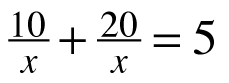3. 5x2 − 6x + 1 = 0

4. x3− 3x2 + 2x = 0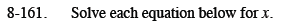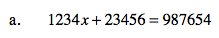x ≈ 781.36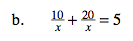Multiply both sides of the equation by x.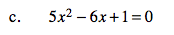Factor and use the Zero Product Property.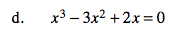x is a common factor. How many solutions do you expect from a cubic equation?